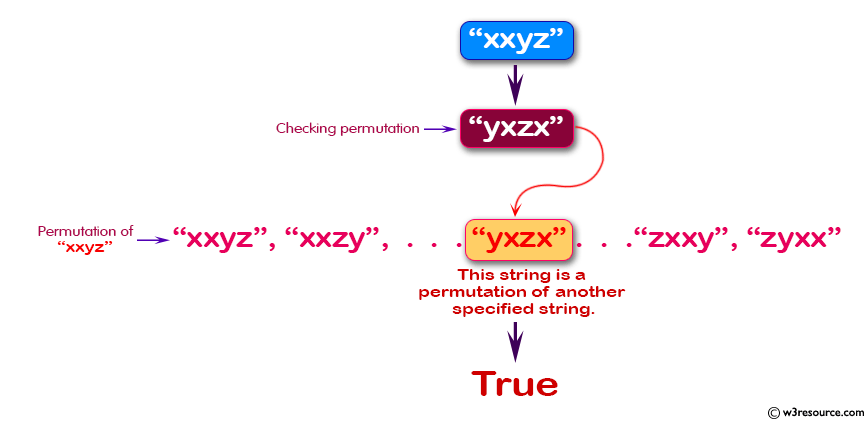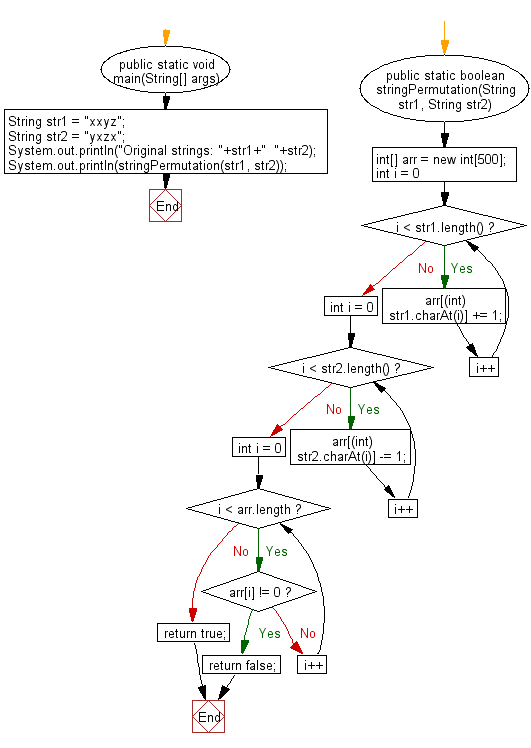﻿ Java: Check if a string is a permutation of another string# Java Exercises: Check if a given string is a permutation of another specified string

## Java Basic: Exercise-149 with Solution

Write a Java program to check if a given string is a permutation of another given string.

Pictorial Presentation:Sample Solution:

Java Code:

``````import java.util.*;
public  class  Solution {
public static void main(String[] args) {
String str1 = "xxyz";
String str2 = "yxzx";
System.out.println("Original strings: "+str1+"  "+str2);
System.out.println(stringPermutation(str1, str2));
}
public static boolean stringPermutation(String str1, String str2) {
int[] arr = new int;
for (int i = 0; i < str1.length(); i++) {
arr[(int) str1.charAt(i)] += 1;
}
for (int i = 0; i < str2.length(); i++) {
arr[(int) str2.charAt(i)] -= 1;
}
for (int i = 0; i < arr.length; i++) {
if (arr[i] != 0) return false;
}
return true;
}
}
```
```

Sample Output:

```Original strings: xxyz  yxzx
true
```

Flowchart:Java Code Editor:

What is the difficulty level of this exercise?

Test your Programming skills with w3resource's quiz.

﻿

## Java: Tips of the Day

countOccurrences

Counts the occurrences of a value in an array.

Use Arrays.stream().filter().count() to count total number of values that equals the specified value.

```public static long countOccurrences(int[] numbers, int value) {
return Arrays.stream(numbers)
.filter(number -> number == value)
.count();
}
```

Ref: https://bit.ly/3kCAgLb